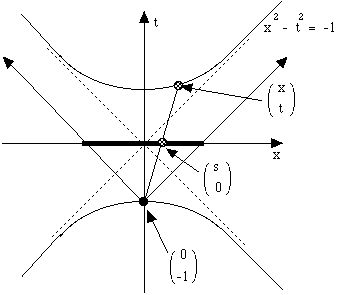# Math 452

Instructor: Bob Connelly

Office: 455 Malott Hall

Telephone: (607) 255-4301 (voice mail)

Math 452, Classical Geometries

This is an introduction to geometry and how it has been moved by a combination of classical Greek influences as well as a more modern desire simply to understand the world as it is seen.

The influence of Euclid's Elements was pervasive in western thought, especially mathematics. The idea is that you should explicitly state what is assumed. One stated one's axioms. But the idea was to minimize such unproved statements. Euclid created a small list of such axioms, but he delayed using the fifth until he was really forced. Did he really need that fifth axiom after all? Could it be deduced it from his other axioms?

Meanwhile, during the Renaissance, artists began to seriously ask just how should one draw a picture that accurately shows what we see? The realization of just how this should be done came almost as a revelation. This led to the development of perspective drawing, and that led to projective geometry. The difference between what is drawn in "correct" perspective and what is not, is striking. The principles perspective are simple, but some of the consequences are not what one might expect.

In a way, projective geometry is an example where one can apply the insight obtained from a simple set of axioms, unlike the situation of Euclid, where there were a very large number of hidden, subtle, complicated, axioms. Projective geometry has just three simple axioms, two are just mirror duals of each other, and the third does not really count. We will use these to show how they provide a great perspective into the nature of geometry, even Euclidean geometry. Projective geometry provides a toy axiomatic system, without a lot of fuss or mess, yet still delivering what is needed.

What do the practical questions of perspective drawing and projective geometry have to do with the esoteric question of whether Euclid's fifth axiom can be deduced from the others? Projective geometry gives one an excellant point of view on how to think think about geometry in general and Euclidean geometry in particular. Indeed, the answer to the question about the fifth axiom, led to the realization that the hyperbolic plane exists. A very natural way to understand the hyperbolic plane (especially with respect to projective geometry) is to think of it as a geometry, but with a distance measured in terms of an indefinite inner product. This in turn is the basis of special relativity.

Along the way in this story, one sees many other interesting and important subjects in mathematics. For example we consider, finite fields related to finite projective planes, orthogonal latin squares used in statistics, the duality of points and lines, the geometry of circles and complex numbers, and the strong analogy between spherical geometry and hyperbolic geometry.

The following picture shows some of the concepts coming from perspective drawing that are based on some ideas of projective geometry. For example, parallel lines project to lines all through the same point, or they project to a collection of parallel lines again. The properties of the projection of a cube, depending on how the cube sits with respect to the viewing plane, lead to some unexpected properties, as well as some very particular constraints, on how one is to draw a cube in realistic perspective.The following picture shows the Minkowskii/Lorenz model of the hyperbolic plane, which is very naturally seen as a hyperboloid of revolution with two sheets. The diagram shows how the projection, viewed along the y-axis, of this surface from the "south pole" onto the horizontal plane gives a pleasant "model" for the hyperbolic plane. This is the model that was promoted by Henri Poincare.Prerequisites: A good course in linear algebra such as Math 221 and an interest in geometry.